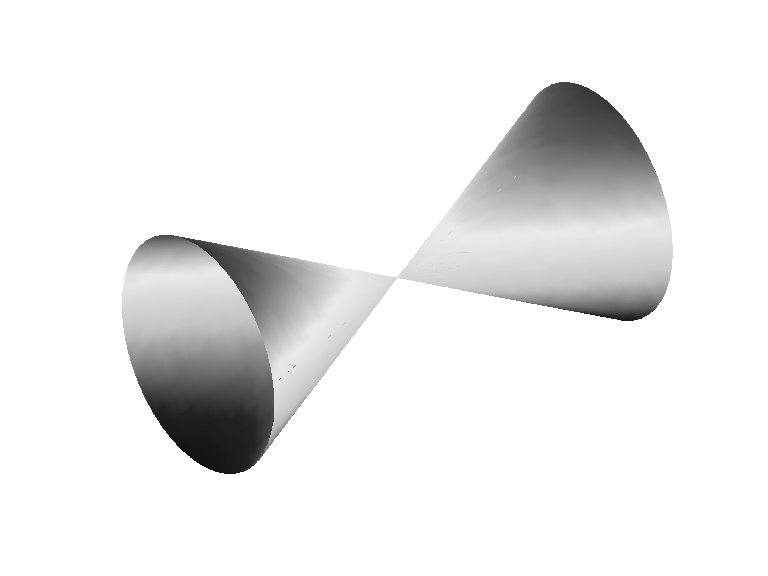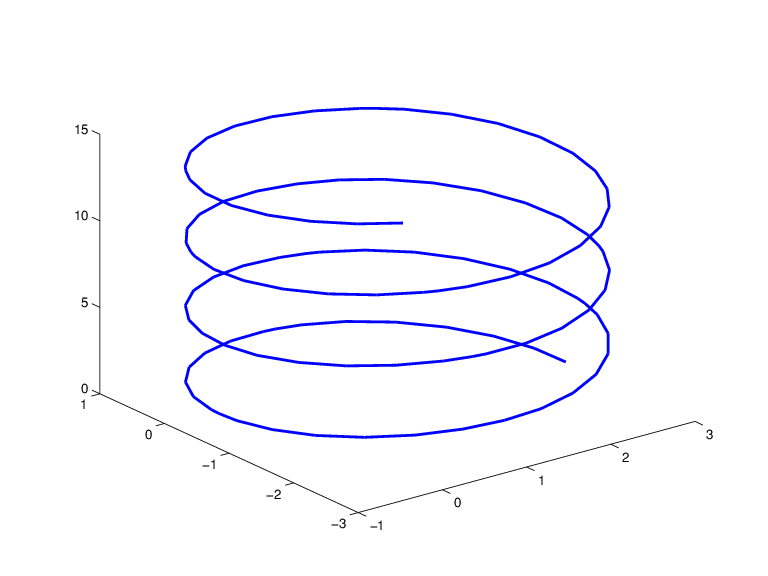# rpmak

Put together rational spline

## Syntax

```rp = rpmak(breaks,coefs) rp = rpmak(breaks,coefs,d) rpmak(breaks,coefs,sizec) rs = rsmak(knots,coefs) rs = rsmak(shape,parameters) ```

## Description

Both `rpmak` and `rsmak` put together a rational spline from minimal information. `rsmak` is also equipped to provide rational splines that describe standard geometric shapes. A rational spline must be scalar- or vector-valued.

`rp = rpmak(breaks,coefs) ` has the same effect as the command `ppmak(breaks, coefs)` except that the resulting ppform is tagged as a rational spline, i.e., as a rpform.

To describe what this means, let R be the piecewise-polynomial put together by the command `ppmak(breaks,coefs)`, and let r(x) = s(x)/w(x) be the rational spline put together by the command `rpmak(breaks,coefs)`. If `v` is the value of R at x, then `v(1:end-1)/v(end)` is the value of r at x. In other words, R(x) = [s(x);w(x)]. Correspondingly, the dimension of the target of r is one less than the dimension of the target of R. In particular, the dimension (of the target) of R must be at least 2, i.e., the coefficients specified by `coefs` must be `d`-vectors with `d `> 1. See `ppmak` for how the input arrays `breaks` and `coefs` are being interpreted, hence how they are to be specified in order to produce a particular piecewise-polynomial.

`rp = rpmak(breaks,coefs,d) ` has the same effect as `ppmak(breaks,coefs,d+1)`, except that the resulting ppform is tagged as being a rpform. Note that the desire to have that optional third argument specify the dimension of the target requires different values for it in `rpmak` and `ppmak` for the same coefficient array `coefs`.

`rpmak(breaks,coefs,sizec) ` has the same effect as `ppmak(breaks,coefs,sizec)` except that the resulting ppform is tagged as being a rpform, and the target dimension is taken to be `sizec(1)-1`.

`rs = rsmak(knots,coefs) ` is similarly related to `spmak(knots,coefs)`, and `rsmak(knots,coefs,sizec)` to `spmak(knots,coefs,sizec)`. In particular, `rsmak(knots,coefs)` puts together a rational spline in B-form, i.e., it provides a rBform. See `spmak` for how the input arrays `knots` and `coefs` are being interpreted, hence how they are to be specified in order to produce a particular piecewise-polynomial.

```rs = rsmak(shape,parameters) ``` provides a rational spline in rBform that describes the shape being specified by the character vector `shape` and the optional additional `parameters`. Specific choices are:

```rsmak('arc',radius,center,[alpha,beta]) rsmak('circle',radius,center) rsmak('cone',radius,halfheight) rsmak('cylinder',radius,height) rsmak('southcap',radius,center) rsmak('torus',radius,ratio) ```

with `1` the default value for `radius`, `halfheight` and `height`, and the origin the default for `center`, and the arc running through all the angles from `alpha` to `beta` (default is `[-pi/2,pi/2]`), and the cone, cylinder, and torus centered at the origin with their major circle in the (x,y)-plane, and the minor circle of the torus having radius `radius*ratio`, the default for `ratio` being `1/3`.

From these, one may generate related shapes by affine transformations, with the help of `fncmb``(rs,transformation)`.

All `fn...` commands except `fnint`, `fnder`, `fndir` can handle rational splines.

## Examples

The commands

```runges = rsmak([-5 -5 -5 5 5 5],[1 1 1; 26 -24 26]); rungep = rpmak([-5 5],[0 0 1; 1 -10 26],1); ```

both provide a description of the rational polynomial r(x) = 1/(x2 + 1) on the interval [-5 .. 5]. However, outside the interval [-5 .. 5], the function given by `runges` is zero, while the rational spline given by `rungep` agrees with 1/(x2 + 1) for every x.

The figure of a rotated cone is generated by the commands

```fnplt(fncmb(rsmak('cone',1,2),[0 0 -1;0 1 0;1 0 0])) axis equal, axis off, shading interp ```

A Rotated Cone Given by a Rational Quadratic SplineA Helix, showing a helix with several windings, is generated by the commands

```arc = rsmak('arc',2,[1;-1],[0 7.3*pi]); [knots,c] = fnbrk(arc,'k','c'); helix = rsmak(knots, [c(1:2,:);aveknt(knots,3).*c(3,:); c(3,:)]); fnplt(helix) ```

A HelixFor further illustrated examples, see NURBS and Other Rational Splines